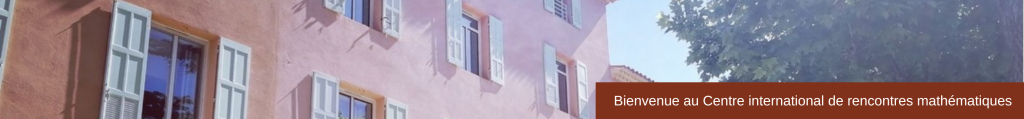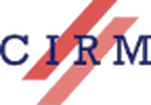CONFERENCE
﻿Analysis and Geometry of Resonances
March 9 – 13, 2015
 Mathematically, quantum resonances appear as discrete eigenvalues of the quantization Ĥ of a Hamiltonian acting on a Hilbert space H on which Ĥ is not self-adjoint, or equivalently as poles of the resolvent (Ĥ − z)−1 of Ĥ on H. Quantum resonances arising from geometric situations –such as from the Laplacian on (asymptotically) hyperbolic manifolds and (locally) symmetric spaces– are linked to interesting geometrical, representation theoretic and analytic objects. In several physical contexts, they provide information about the time evolution of propagation of states with certain regularity properties or decay at infinity. They also come naturally in trace formulas relating geometric invariants and spectral invariants (like Selberg trace formula). They are therefore important data relating classical and quantum dynamics. The questions are their existence, distribution (counting functions, spectral gap), stability under perturbations. Another aspect of resonances is linked to hyperbolic dynamics. Modern microlocal techniques allow to show that the vector field X generating a smooth Anosov flow φt on a compact manifold has a resolvent (X − z)−1 which extends meromorphically in z ∈ C on certain anisotropic Sobolev spaces associated to the unstable/stable foliations. The poles are called classical resonances. Their location (like obtaining a spectral gap) is fundamental to obtain precise description of the long time behaviour of correlations. Classical resonances also appear in trace formulas relating closed orbits of the flow and spectrum of X. The last years have seen considerable progress in understanding the geometry and analysis of classical and quantum resonances, but many interesting (and difficult) questions in the subject are open. The purpose of this workshop is to gather researchers working on the different aspects of the geometric theory of resonances (spectral geometry, representation theory, harmonic or microlocal analysis, analytic number theory, mathematical physics) to present their latest results, share their points of view and ideas, strengthen or promote interactions. Scientific & Organizing Committee Colin Guillarmou (ENS Paris)Joachim Hilgert (University of Paderborn)Angela Pasquale (Université de Lorraine)Tomasz Przebinda (University of Oklahoma) Speakers Viviane Baladi (ENS Paris)  The spectrum of Sinai billiard flows Yannick Bonthonneau (ENS Paris)  Resonances-free regions for cusp manifolds David Borthwick (Emory University, Atlanta)  Symmetry factorization of Selberg zeta functions and distributions of resonances Nicolas Burq (Université Paris-Sud) Euclidean scattering, resolvent estimates and evolution PDE’s Gilles Carron (Université de Nantes)  Riesz transform on manifolds with quadratic curvature decay  Kiril Datchev (Purdue University) Semiclassical resolvent bounds in general trapping situations Semyon Dyatlov (Massachusetts Institute of Technology) A microlocal toolbox for hyperbolic dynamics Frédéric Faure (Université Joseph Fourier) Peter Hintz (Stanford University) Asymptotics for the wave equation on differential forms on Kerr-de Sitter space Lizhen Ji (University of Michigan) Geometry and analysis of locally symmetric spaces of infinite volume Roberto Miatello (University of Cordoba) Resolvent and lattice points on symmetric spaces of negative curvature Werner Müller (University of Bonn) Resonances for the Laplacian on locally symmetric spaces of finite volume Frédéric Naud (Université d’Avignon) Nodal lines and domains for Eisenstein series on surfaces Martin Olbrich (Université du Luxembourg) Transfer operators, resonances, and group cohomology for hyperbolic manifolds of infinite volume Aprameyan Parthasarathy (University of Paderborn) Scattering poles for rank one symmetric spaces Peter Perry (University of Kentucky) Scattering resonances on hyperbolic manifolds as a model of chaotic scattering Anke Pohl (University of Göttingen) Transfer operators for Riemann surfaces of finite and infinite area with cuspidal ends  Mark Pollicott (University of Warwick) Zeta functions, decay of correlations and resonances  Pablo Ramacher (Philipps-University Marburg) Quantum ergodicity and symmetry reduction (pdf) Alexander Strohmaier (Loughborough University) ﻿Resonances and symmetric spaces﻿ Duc Viet Vu (Institut de Mathématique de Jussieu-Paris Rive Gauche) Asymptotic number of scattering resonances for generic Schrödinger operators Polina Vytnova (University of Warwick) Estimating fast dynamo growth rate using Zeta functions Tobias Weich (Philipps-University Marburg) Resonance chains on Schottky surfaces Chengbo Zhu (National University of Singapore) Conservation relations for local theta correspondence Maciej Zworski (University of California, Berkeley) Resonances as viscosity limits